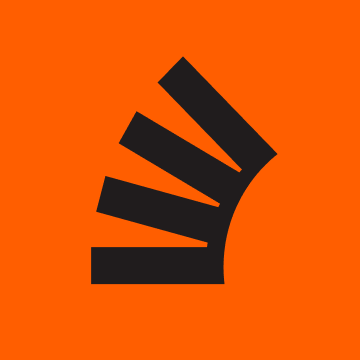# Add a button with functionality in c++ using Visual Studio

I am a newbie to c++ and the IDE I am using is Visual Studio ’22. I have written a code to detect a face (eyes and mouth too) and save the roi to a folder on the pc. Now what it does can be thought of as an auto-capture of the roi as soon as the face is detected.

I now want to create the function for “force capture”, for which I will need to have a button and add pretty much the same code I wrote for auto-capture to give it functionality.

How do I add the button and make it perform its task?

I found related answers but they use Qt not sure how to apply that here.

Thanks a ton! Really need help.

``````#include <opencv2/opencv.hpp>
#include <opencv2/dnn/dnn.hpp>
#include "opencv2/face.hpp"
#include "opencv2/imgproc.hpp"
#include "opencv2/imgcodecs.hpp"
#include "opencv2/highgui.hpp"
#include "opencv2/objdetect.hpp"
#include <iostream>
#include <opencv2/imgproc/types_c.h>
//file handling
#include <fstream>
#include <sstream>

using namespace cv;
using namespace std;
using namespace ml;
using namespace cv::face;

//(3) Global variables
Ptr<Facemark> facemark; //mark detection
CascadeClassifier faceDetector, mouth, eye, eye1; //face detection
string name, filename;

void process(Mat img, Mat imgcol) {
vector<Rect> faces;
faceDetector.detectMultiScale(img, faces);

Mat imFace;

if (faces.size() != 0) {

for (size_t i = 0; i < faces.size(); i++)
{
cv::rectangle(imgcol, faces[i], Scalar(255, 0, 0));
imFace = imgcol(faces[i]);
resize(imFace, imFace, Size(imFace.cols * 5, imFace.rows * 5));
faces[i] = Rect(faces[i].x = 0, faces[i].y = 0, faces[i].width * 5,
(faces[i].height) * 5);
}
vector< vector<Point2f> > shapes;
//vector < Rect > measures;
if (facemark->fit(imFace, faces, shapes))   //fiiting predef shapes in faces// // imface is the size of faces
{
for (unsigned long i = 0; i < faces.size(); i++) {
for (unsigned long k = 0; k < shapes[i].size(); k++) {
cv::circle(imFace, shapes[i][k], 5, cv::Scalar(0, 0, 255), FILLED);
}
}
}
namedWindow("Detected_shape");
imshow("Detected_shape", imFace);
waitKey(5);
}

else {
cout << "Faces not detected." << endl;
}
}

int main()
{
facemark = FacemarkLBF::create();

cout << "Loaded model" << endl;

Mat frame, grayframe, testframe, faceROI;
int x_axis, y_axis;
namedWindow("Detecting");

VideoCapture cap(0); //1 for diff cam

while (1)
{
{
cout << "an error while taking the frame from cap" << endl;
}

//face
vector<Rect> faces;

Mat frame_gray;
Mat crop;
Mat res;
Mat gray;
string text;
stringstream sstm;

cvtColor(frame, grayframe, CV_BGR2GRAY);
equalizeHist(grayframe, testframe);
faceDetector.detectMultiScale(testframe, faces, 1.1, 3, CASCADE_SCALE_IMAGE, Size(30, 30));

Rect roi_b;
Rect roi_c;
size_t ic = 0;
int ac = 0;
size_t ib = 0;
int ab = 0;

for (int ic = 0; ic < faces.size(); ic++)
{
roi_b.x = faces[ib].x;
roi_b.y = faces[ib].y;
roi_b.width = (faces[ib].width);
roi_b.height = (faces[ib].height);
crop = frame(roi_b);
resize(crop, res, Size(128, 128), 0, 0, INTER_LINEAR);
cvtColor(crop, gray, COLOR_BGR2GRAY);
stringstream ssfn;
filename = "C:\Users\Hp\Desktop\Faces\";
ssfn << filename.c_str() << name <<"_"<< roi_b.width<<"_"<< roi_b.height << ".jpg";
filename = ssfn.str();
imwrite(filename, res);
rectangle(frame, faces[ic], Scalar(255, 0, 255), 2, 8, 0);
Mat face = frame(faces[ic]);
cvtColor(face, face, CV_BGR2GRAY);

//mouth
vector <Rect> mouthi;
mouth.detectMultiScale(face, mouthi);
for (int k = 0; k < mouthi.size(); k++)
{
Point pt1(mouthi.x + faces[ic].x, mouthi.y + faces[ic].y);
Point pt2(pt1.x + mouthi.width, pt1.y + mouthi.height);
rectangle(frame, pt1, pt2, Scalar(255, 0, 0), 1, 8, 0);
}

//eyes
faceROI = frame(faces[ic]);//Taking area of the face as Region of Interest for eyes//

vector<Rect>eyes;//declaring a vector named eyes//
eye.detectMultiScale(faceROI, eyes, 1.1, 3, 0 | CASCADE_SCALE_IMAGE, Size(5, 5)); //detect eyes in every face//
/*eye1.detectMultiScale(faceROI, eyes, 1.1, 3, 0 | CASCADE_SCALE_IMAGE, Size(5, 5));*/

for (size_t j = 0; j < eyes.size(); j++)
{ //for locating eyes//

Point center(faces[ic].x + eyes[j].x + eyes[j].width * 0.5, faces[ic].y + eyes[j].y + eyes[j].height * 0.5);//getting the centers of both eyes//
int radius = cvRound((eyes[j].width + eyes[j].height) * 0.25); //declaring radius of the eye enclosing circles//
circle(frame, center, radius, Scalar(255, 0, 0), 1, 8, 0);//drawing circle around both eyes//
x_axis = eyes[j].x;//storing x axis location of eyes in x_axis//
y_axis = eyes[j].y;//storing y axis location of eyes in y_axis//
cout << "Position of the eyes is:" << "(" << x_axis << "," << y_axis << ")" << endl;//showing co-ordinate values//
}

}
sstm << "Crop area size: " << roi_b.width << "x" << roi_b.height << " Filename: " << filename;
text = sstm.str();
if (!crop.empty()) {
imshow("detected", crop);
}
else destroyWindow("detected");

cout << "Namen";
cin >> name;

Mat img;   //image containers
Mat imgbw;
cap >> img;   //image from webcam
resize(img, img, Size(460, 460), 0, 0, INTER_LINEAR_EXACT);
cvtColor(img, imgbw, COLOR_BGR2GRAY);
process(imgbw, img);
imshow("Detecting", frame);
if (waitKey(30) == 27) {
break;
}
}
return 0;
}
``````

This site uses Akismet to reduce spam. Learn how your comment data is processed.

Scroll to Top